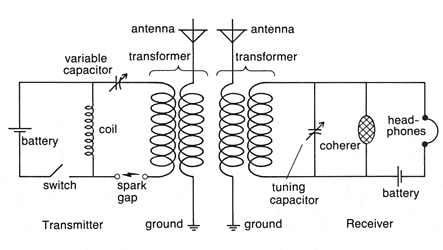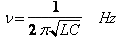# A little help with this circuit

manual
hello,
i would like to know something for this circuiti am wondering how can i calculate the resonant frequency for this circuit.
as i know, i can calculate the resonant frequency using the following equation:my problem is if the coil = 2 microhenry for the sending circuit and the variable capacitor is set to 1 nanofarad for the sending circuit and receiving circuit. how would the transformer for the antenna effect my calculations? will it change the resonant frequency?

i am looking forward to knowing how can i calculate the frequency for this circuit if the "coil" is 2 microhenry and the primary + the secondary is 1 microhenry for the transformer in the transmitter and receiver circuit (the capacitor is 1 nanofarad for transmitter and receiver circuit)

With my best regards.

#### Attachments

Last edited:

You would have to know quite a bit more about the circuit components, but if the antenna was resonant and produced a resistive load for the tuned circuits, it would affect the Q of the tuned circuits, but not the frequency.

In each case the primary of the transformer for the antenna is the main inductance for the tuned circuit.

Just the presence of the secondary winding does not affect the inductance of the primary much, but connecting an antenna that was mostly capacitive would affect the resonant frequency of the tuned circuit.

The receive tuned circuit would resonate at about 3.56 MHz with the components you list.

That circuit would not have been used for 90 years or so. Spark gaps and coherers have been replaced a long time ago.

manual
thanks for replay,

by the way is this a LC series or parallel circuit?
it makes me confused.

In each case the primary of the transformer for the antenna is the main inductance for the tuned circuit.
what about the coil? how it will effect the inductance in the sending circuit?

The transmitter uses a series tuned circuit and the receiver uses a parallel tuned circuit.

Battery power to the "coil" is switched rapidly by the "switch" and this generates a high voltage across it and this contains a lot of high frequency harmonics.

The tuned circuit (consisting of the capacitor and the primary of the transformer) act to filter out some of this harmonic energy so that a relatively small range of frequencies would be transmitted.

By today's standards, they were horrible wideband noise makers

manual
thanks for replay again, bro ;)

The tuned circuit (consisting of the capacitor and the primary of the transformer) act to filter out some of this harmonic energy so that a relatively small range of frequencies would be transmitted.

So the primary of the transformer must has inductance of 2 microhenry to get about 3.56 MHz and the 2 microhenry coil won't effect the frequency even if i changed it to 3 microhenry and set the primary of the transformer for the transmitter circuit to 2 microhenry (the capacitor is 1 nanofarad for each circuit).

So the primary and the secondary of the transformer in the receiving circuit must be 2 microhenry to get the range of frequencies what the transmitter circuit is sending (3.56 MHz).

am i right?

Last edited:
You would need to know the actual values to work out the resonance frequencies.

I would guess that the "coil" has a much greater inductance than the coupling transformer primary, but spark transmitters were a little before my time.

The circuit looks very much like a Kettering ignition system and the antenna transformer is able to extract a little of the energy flowing in the circuit and filter it so that is is comparatively narrow banded in its frequency range.

I hope you are not thinking of building one of these?

manual
i am not going to build it, but i am looking forward to knowing how can i calculate the frequency for this circuit if the "coil" is 2 microhenry and the primary + the secondary is 1 microhenry for the transformer in the transmitter and receiver circuit (the capacitor is 1 nanofarad for transmitter and receiver circuit) Because i am reading and learning about LC + RLC circuits and this one making me confused when the transformer combined with the coil in the transmitter and receiver circuit.

Last edited: